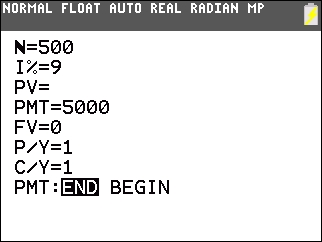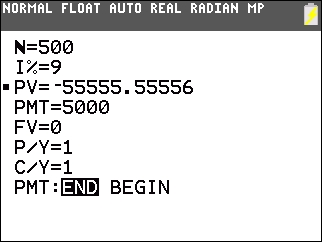# Knowledge Base

## Solution 34919: Calculating the Present Value of a Perpetuity (Infinite Annuity) on the TI-83 Plus and TI-84 Plus Family of Graphing Calculators.

### How can I calculate the present value of a perpetuity (infinite annuity) on the TI-83 Plus and TI-84 Plus family of graphing calculators?

Sometimes when dealing with annuities, there are those that pay forever instead of for a specific period of time. Hence, they are called perpetuities or infinite annuities. Since these units do not have a way to specify an infinite number of periods for N, the calculation involves the fact that the present value of a cash flow far enough into the future is going to be approximately $0. This means that futuristically the cash flows no longer add anything to the present value. Therefore, selecting a large number of payments yields a close approximation to a perpetuity. For example, the payment is$5,000 per year and the interest rate is 9% annually. Since it is a perpetuity, the user can select 500 as N. First, access the TVM solver by pressing [APPS] [ENTER] [ENTER].Next, place the cursor next to PV and press [ALPHA] [SOLVE] to compute the present value:The present value is \$-55,555.56.

Please Note: There is not a way to find the future value of a perpetuity because the cash flows never end.

Please see the TI-83 Plus and TI-84 Plus Family guidebooks for additional information.